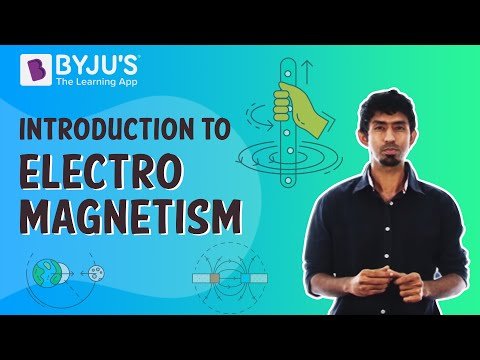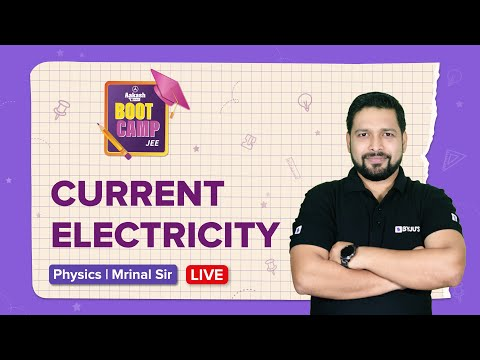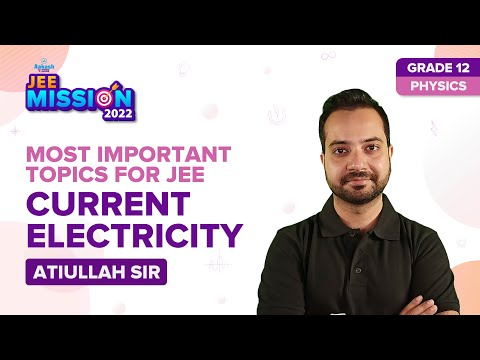Checkout JEE MAINS 2022 Question Paper Analysis : Checkout JEE MAINS 2022 Question Paper Analysis :

# Current Electricity Questions

The flow of electrons in a circuit is known as current electricity. The carriers of electric current are electrons. The current in a circuit is due to the flow of electrons, and the carriers of electric current are electrons. Therefore, it can be concluded that the type of current that flows through a circuit is electric current.

Ampere is the SI unit of current. If one coulomb of charge flows through the wire per second, then the current through a wire is called one ampere.

The current that flows from positive potential to negative potential, that is, from higher potential to lower potential, is called conventional current. The direction of electric current will be taken as the direction of motion of positive charges.

Static electricity

When the positive and negative charges are separated, the phenomenon of static electricity arises. So, an electric charge that builds up on the surface of substances or materials is known as static electricity. Until the charges are grounded or discharged, they remain static. Due to friction, this type of electricity is formed.

### Direct current

The constant flow of electrons from a region of high electron density to a region of low electron density is known as direct current. Hence, it can be concluded that the current electricity whose direction remains the same is known as direct current. Many household appliances use DC that involves a battery.

Alternating current

Alternating current is the current electricity that keeps changing the direction of the charge flow and is bidirectional in nature. A sinusoidally fluctuating current and voltage that reverses directions is the reason behind this bidirectionality, due to which a periodic back and forth motion for the current is created. Alternating current is supplied in the electrical outlets at our homes and industries.

## Important Questions on Current Electricity

1) The SI unit of current is _____.

a) C (Coulomb)

b) A (Ampere)

d) N (Newton)

Correct Option: (b)

Explanation: Ampere is the SI unit of current. If one coulomb of charge flows through the wire per second, then the current through a wire is called one ampere.

2) Which among the following is the accurate definition of conventional current?

a) Conventional current flows from lower potential to higher potential

b) Conventional current is the current which remains static

c) Conventional current is created by the flow of ions

d) Conventional current flows from higher potential to lower potential

Correct Option: (d)

Explanation: The current that flows from positive potential to negative potential, that is, from higher potential to lower potential, is called conventional current. The direction of electric current will be taken as the direction of motion of positive charges.

3) Which type of current flows through a circuit?

a) Potential current

b) Static current

c) Electric current

d) Dynamic current

Correct Option: (c)

Explanation: The carriers of electric current are electrons. The current in a circuit is due to the flow of electrons, and the carriers of electric current are electrons. Therefore, it can be concluded that the type of current that flows through a circuit is electric current.

4) The material which is suitable for making standard resistors is _____.

a) Copper

b) Silver

c) Constantan

d) Germanium

Correct Option: (c)

Explanation: For making standard resistance coils, alloys like constantan or manganin are used because they have a very small temperature coefficient and high resistivity values.

5) The current carriers are free electrons in electrolytes and ionic crystals. Is it true or false?

a) True

b) False

Correct Option: (b)

Explanation: The current carriers are positive and negative ions in electrolytes and ionic crystals. On the other hand, electrons carry current in most substances, like metals.

6) The study of electric charges in motion is called _____.

a) Charge mobility

b) Electronic mobility

c) Static electricity

d) Current electricity

Correct Option: (d)

Explanation: The study of electric charges in motion is called current electricity because the study of motion or dynamics of charges establishes an electric current.

7) Which among the following elements is the most conductive element?

b) Aluminium

c) Silver

d) Iron

Correct Option: (c)

Explanation: One of the most electrically conductive elements is silver, and the reason behind it is that the electrons in silver can move more freely as compared to any other element.

8) What is static electricity?

When the positive and negative charges are separated, the phenomenon of static electricity arises. So, an electric charge that builds up on the surface of substances or materials is known as static electricity. Until the charges are grounded or discharged, they remain static. Due to friction, this type of electricity is formed.

### 9) What is direct current?

The constant flow of electrons from a region of high electron density to a region of low electron density is known as direct current. Hence, it can be concluded that the current electricity whose direction remains the same is known as direct current. Many household appliances use DC that involves a battery.

### 10) What is alternating current?

Alternating current is the current electricity that keeps changing the direction of the charge flow and is bidirectional in nature. A sinusoidally fluctuating current and voltage that reverses directions is the reason behind this bidirectionality, due to which a periodic back and forth motion for the current is created. Alternating current is supplied in the electrical outlets at our homes and industries.

### Practice Questions

1) Define 1 ampere.

2) What is current?

3) State and explain Ohm’s law.

4) Define potential difference.

5) What is resistance?

## Suggested Videos

#### Introduction to Electromagnetism – BYJU’S#### Combination of Resistors: Current Electricity Class 12 Physics (Ch-3) Questions | JEE Main 2022 Exam#### Current Electricity Class 12 Physics (Ch-3) | JEE Main 2022 Important Topics | JEE 2022 Exam Prep#### Electric Field Lines | PHYSICS | NEET | Concept of the Day | Mrinal SirStay tuned to BYJU’S and Fall in Love with Learning!